# Halfedge Data Structures

## Introduction

A halfedge data structure is an edge-centered data structure capable of maintaining incidence informations of vertices, edges and facets, for example for planar maps, polyhedra, or other orientable, two-dimensional surfaces embedded in arbitrary dimension. Each edge is decomposed into two halfedges with opposite orientations. One incident facet and one incident vertex are stored in each halfedge. For each facet and each vertex one incident halfedge is stored. Reduced variants of the halfedge data structure can omit some of these informations, for example the halfedge pointers in vertices or the storage of vertices at all.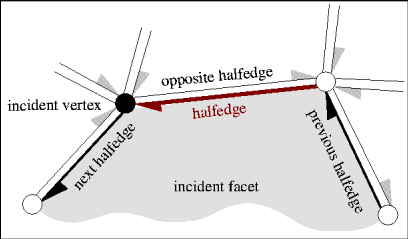The data structure provided here is known as the FE-structure [Wei85], as halfedges [Män88, BFH95] or as the doubly connected edge list (DCEL) [dBvKOS97], although the original reference for the DCEL [MP78] describes a different data structure. We continue naming it halfedge data structure (HDS). This data structure can also be seen as one of the variants of the quad-edge data structure [GS85], reduced to support only orientable surfaces. In general, the quad-edge data structure has a richer modeling space and can represent non-orientable 2-manifolds. The dual and the primal graph are symmetrically represented; the same algorithms can be applied to the dual graph as well as to the primal graph. This implies a lack of static type checking to distinguish between facets and vertices. Even though halfedges are similar to the winged edge data structure [Bau75] or the original reference for the DCEL [MP78], they are not equivalent, since the traversal algorithms are forced to search for certain incidences where the information is directly available in the halfedge data structure. An overview and comparison of these different data structures together with a thorough description of the design implemented here can be found in [Ket98].

### Design Overview

The design strictly separates topology and geometry. Vertices, halfedges and facets carry both kinds of information. The Halfedge_data_structure acts as a container class and stores all items and manages their incidence relations. For example the Polyhedron from Chapteruses the Halfedge_data_structure and adds geometric operations. It imposes further restrictions on the data structure, for example, that an edge always has two distinct endpoints.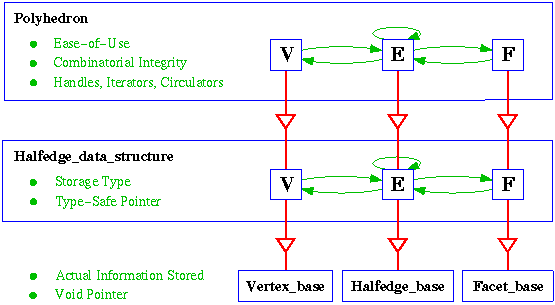Figure: Responsibilities of the different layers in the halfedge data-structure design.

Figureoffers a closer look at the design using Polyhedron as example. Note that Halfedge_data_structure, Vertex_base, Halfedge_base and Facet_base are concepts, where each can have multiple models. There are many different possibilities for vertices, edges and facets. Currently, two different models are provided for the Halfedge_data_structure. Many combinations are possible and result in a different Polyhedron. We make use of the implicit instantiation of template classes in C++. The requirement set for a model consists of a mandatory part that every model must comply with and certain optional parts a model must only comply with if the corresponding functionality is actually used. For example, a vertex is allowed to be empty. If we want to use it for the polyhedral surface, then the normal vector computation imposes the additional requirements that the vertex must contain a three-dimensional point and gives access to it with the member function point().

The responsibilities of the base classes for vertices, halfedges and facets are the actual storage of the incidences in terms of void-pointers, the geometry and other attributes. Especially the storage of incidences with void-pointers allows, for example, the facet class to be exchanged without rewriting the halfedge class. The advantage of strong type checking will be reestablished in the next layer. Implementations for vertices, edges and facets are provided that fulfill the minimal set of requirements. They can be used as base classes for own extensions. Richer implementations are provided as defaults; for polyhedra they provide a three-dimensional point in the vertices and a plane equation in the facets.

The Halfedge_data_structure is responsible for the storage organization of the vertices, halfedges and facets. Currently, implementations are provided that use a bidirectional list or an STL std::vector internally. The Halfedge_data_structure derives new classes for vertices, halfedges and facets. They replace the void-pointer incidence information with type-safe pointers at the interface. Additional information besides the incidence information simply stays unaffected and will be inherited.

Different models are possible for the Halfedge_data_structure (two are available right now). Thus the set of requirements for the Halfedge_data_structure is kept small. To support the implementation of high-level operations, a helper class Halfedge_data_structure_decorator is provided, which is not shown in Figurebut would be placed at the side of the Halfedge_data_structure, since it broadens that interface but does not hide it. It adds Euler operations and adaptive functionality. For example, if the prev() function is not provided for halfedges, a find_prev() function searches the previous halfedge along the facet. If the prev() function is available, the find_prev() function simply calls it. This distinction is resolved at compile time with a technique called compile-time tags, similar to iterator tags in [SL95].

The Polyhedron (Chapter) adds an ease-of-use layer in terms of high-level functions, high-level concepts for accessing the items, i.e. handles, iterators and circulators (pointers are no longer visible at this interface), and the protection of the combinatorial integrity. It derives new vertices, halfedges and facets to provide the handles and hide the pointers.

### Organization of this Chapter

Sectiondescribes the requirements a Halfedge_data_structure with its three local classes for vertices, halfedges and facets must fulfill. Sectionnames the models currently available. These models make use of the Vertex_base, Halfedge_base and Facet_base concepts, whose requirements are stated in Section. The predefined base classes are described in Section. The helper class Halfedge_data_structure_decorator follows in Section. Several examples of halfedge data structures in Sectionconclude the chapter.

• Class declaration of Facet.

## Models of Halfedge_data_structure

Four models are currently provided for the Halfedge_data_structure concept in CGAL. Two generic models are parameterized with models of the Vertex_base, Halfedge_base and Facet_base concepts: Halfedge_data_structure_using_list manages the items with a doubly-linked list, and Halfedge_data_structure_using_vector manages the items internally with an STL std::vector. Two further models provide default solutions based on the generic model using lists. The model Halfedge_data_structure_default implements all incidences and allows to choose a point type for vertices. Halfedge_data_structure_polyhedron_default_3 is prepared for polyhedral surfaces. It implements again all incidences and stores a three-dimensional point in vertices and a plane equation in facets, see Sectionfor details.

## Requirements for Vertex_base, Halfedge_base and Facet_base

Two predefined models of the Halfedge_data_structure concept make use of the concepts of Vertex_base, Halfedge_base and Facet_base, whose requirements are defined in the following subsections. The incidence relations and the mandatory and optional member functions are summarized in Figure.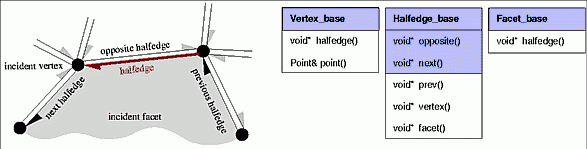Figure: The three concepts Vertex_base, Halfedge_base, and Facet_base for the halfedge data structure. Member functions with shaded background are mandatory. The others are optionally supported.

## Models of Vertex_base, Halfedge_base and Facet_base

CGAL currently provides the following models for the concepts of Vertex_base, Halfedge_base and Facet_base. The first set of models implements the minimal set of requirements demanded for the concepts. The second set provides all described incidences and a point type for vertices. Another model for the Facet_base concept is tailored for polyhedral surfaces and includes a plane equation, see Section. These models can be used directly or they can serve as base classes for further developments.

#include <CGAL/Halfedge_data_structure_bases.h>

 class Vertex_min_base; defines the minimal vertex functionality with no data at all. template class Vertex_max_base; defines the maximal vertex functionality including halfedge pointer and a template parameter for the point. class Halfedge_min_base; defines the minimal halfedge functionality with next and opposite pointers. class Halfedge_max_base; defines the maximal halfedge functionality including previous, vertex and facet pointers. class Facet_min_base; defines the minimal facet functionality with no data at all. class Facet_max_base; defines the maximal functionality for facets including halfedge pointer.

Halfedge_data_structure_using_list<V,H,F> and
Halfedge_data_structure_using_vector<V,H,F>.

## Examples of Halfedge Data Structures

### The Default Halfedge Data Structure

The default halfedge data structure from Sectionexpects a point as template argument, which we choose to be an int for simplicity. The example program defines a halfedge data structure and a decorator, see Sectionfor the decorator. The program creates a loop, consisting of two halfedges, one vertex and two facets, and checks its validity.

```// hds_prog_default.C
// ------------------------------------------------
#include <CGAL/Halfedge_data_structure_default.h>
#include <CGAL/Halfedge_data_structure_decorator.h>

typedef int                                           Point;
typedef CGAL::Halfedge_data_structure_default<Point>  HDS;
typedef CGAL::Halfedge_data_structure_decorator<HDS>  Decorator;

int main() {
HDS hds;
Decorator decorator;
decorator.create_loop( hds);
CGAL_assertion( decorator.is_valid( hds));
return 0;
}
```

### A Minimal Halfedge Data Structure

The following program declares a minimal structure HDS using the minimal bases provided in Sectionand a list-bases halfedge data structure from Section. The result is a data structure maintaining only halfedges with next and opposite pointers. No vertices or facets are stored. The data structure models an undirected graph.

```// hds_prog_graph.C
// -------------------------------------------------
#include <CGAL/Halfedge_data_structure_bases.h>
#include <CGAL/Halfedge_data_structure_using_list.h>
#include <CGAL/Halfedge_data_structure_decorator.h>

typedef CGAL::Halfedge_data_structure_using_list <
CGAL::Vertex_min_base, CGAL::Halfedge_min_base, CGAL::Facet_min_base> HDS;
typedef CGAL::Halfedge_data_structure_decorator<HDS>  Decorator;

int main() {
HDS hds;
Decorator decorator;
decorator.create_loop( hds);
CGAL_assertion( decorator.is_valid( hds));
return 0;
}
```

### The Default with a Vector Instead of a List

The default halfedge data structure uses a list internally and the maximal base classes. Assuming we have a point type Point we can declare an alternative data structure HDS using the same base classes but the vector storage from Section. Note that for the vector storage the size of the halfedge data structure must be reserved beforehand, either with the constructor as shown in the example or with the reserve() member function.

```// hds_prog_simple.C
// ---------------------------------------------------
#include <CGAL/Halfedge_data_structure_bases.h>
#include <CGAL/Halfedge_data_structure_using_vector.h>
#include <CGAL/Halfedge_data_structure_decorator.h>

using namespace CGAL;

typedef int  Point;
typedef Halfedge_data_structure_using_vector <
Vertex_max_base<Point>, Halfedge_max_base, Facet_max_base> HDS;
typedef Halfedge_data_structure_decorator<HDS>  Decorator;

int main() {
HDS hds(1,2,2);
Decorator decorator;
decorator.create_loop( hds);
CGAL_assertion( decorator.is_valid( hds));
return 0;
}
```

### Example Adding Color to Facets

This example re-uses the base class available for facets and adds another member variable color. The facet in the halfedge data structure and data structures building upon that will inherit the new member variable, as the main function indicates. The same can be done with any kind of functionality and any of the three items; vertices, halfedges or facets. However, note that additional pointers to vertices, halfedges, and facets cannot be introduced this way, since they are only maintained as void* pointers at this level. The internal classes, which are responsible for the type safe pointers, must be extended to achieve this.

```// hds_prog_color.C
// -------------------------------------------------
#include <CGAL/basic.h>
#include <CGAL/IO/Color.h>
#include <CGAL/Halfedge_data_structure_bases.h>
#include <CGAL/Halfedge_data_structure_using_list.h>

using namespace CGAL;

// A facet with a color member variable.
struct My_facet : public Facet_max_base {
Color color;
};

typedef int  Point;
typedef Halfedge_data_structure_using_list <
Vertex_max_base<Point>, Halfedge_max_base, My_facet> HDS;

int main() {
HDS hds;
My_facet* f = hds.new_facet();
f->color = RED;
return 0;
}

```

### Example Extending the Minimal Halfedge Base with a Previous Pointer

The minimal halfedge data structure above can be extended to a data structure also supporting a previous pointer for halfedges. We replace the Halfedge_min_base with our own Halfedge_base derived from the Halfedge_min_base.

```  class My_halfedge : public Halfedge_min_base {
void* prv;
public:
typedef  Tag_true   Supports_halfedge_prev;
void*       prev()                    { return prv;}
const void* prev() const              { return prv;}
void        set_prev( void* h)        { prv = h;}
};
```

### Example Using own Halfedge Structure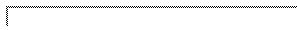The halfedge data structure as presented here is not as space efficient as for example the winged edge data structure [Bau75], the DCEL [MP78] or variants of the quad-edge data structure [GS85]. On the other side they do not need any search operations during traversals. A comparison can be found in [Ket98].

Computation time versus memory space can also be traded with the halfedge data structure design as presented here. We will use traditional C techniques like type casting and make the assumption that pointers (especially those from malloc or new) points to even addresses. The idea is as follows: The halfedge data structure allocate halfedges pairwise. Concerning the vector based data structure this implies that the absolute value of the difference between a halfedge and its opposite halfedge is always one with respect to C pointer arithmetic. We can replace the opposite pointer by a single bit coding the sign of this difference. We will store this bit as the least significant bit together with the next halfedge pointer. The example also omits the previous pointer. What remains are three pointers per halfedge. The same solution can be applied to the list based polyhedron traits class with the difference that the SIZE constant must reflect the additional pointers needed for the list management. They are added internally of the halfedge data structure using the base class In_place_list_base<My_halfedge>.

```// hds_prog_compact.C
// ---------------------------------------------------
#include <CGAL/Halfedge_data_structure_bases.h>
#include <CGAL/Halfedge_data_structure_using_vector.h>
#include <CGAL/Halfedge_data_structure_decorator.h>

using namespace CGAL;

// Define a new halfedge class.
class My_halfedge {
protected:
size_t  nxt;
void*   v;
void*   f;
public:
typedef Tag_false Supports_halfedge_prev;
typedef Tag_true  Supports_halfedge_vertex;
typedef Tag_true  Supports_halfedge_facet;

My_halfedge() : nxt(0), f(NULL) {}

void*       opposite()       {
const size_t SIZE = sizeof( My_halfedge);
if ( nxt & 1)
return (char*)this + SIZE;
return (char*)this - SIZE;
}
const void* opposite() const {
const size_t SIZE = sizeof( My_halfedge);
if ( nxt & 1)
return (const char*)this + SIZE;
return (const char*)this - SIZE;
}
void*       next()           { return (void*)(nxt & (~ size_t(1)));}
const void* next() const     { return (void*)(nxt & (~ size_t(1)));}

void*       vertex()         { return v;}
const void* vertex() const   { return v;}

void*       facet()          { return f;}
const void* facet() const    { return f;}

bool is_border() const       { return f == NULL;}

void  set_opposite( void* g) {
const size_t SIZE = sizeof( My_halfedge);
char* h = (char*)g;
CGAL_assertion( size_t( abs( h - (char*)this)) == SIZE);
if ( h > (char*)this)
nxt |= 1;
else
nxt &= (~ size_t(1));
}
void  set_next( void* h)     {
CGAL_assertion( ((size_t)h & 1) == 0);
nxt = ((size_t)(h)) | (nxt & 1);
}
void  set_vertex( void* _v)  { v = _v;}
void  set_facet( void* _f)   { f = _f;}
};

typedef int  Point;
typedef Halfedge_data_structure_using_vector <
Vertex_max_base<Point>, My_halfedge, Facet_max_base> HDS;
typedef Halfedge_data_structure_decorator<HDS>  Decorator;

int main() {
HDS hds(1,2,2);
Decorator decorator;
decorator.create_loop( hds);
CGAL_assertion( decorator.is_valid(hds));
return 0;
}
```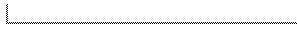### Example Using the Halfedge Iterator

Three edges are created in the default halfedge data structure. The halfedge iterator is used to count the halfedges.

```// hds_prog_halfedge_iterator.C
// ------------------------------------------------
#include <CGAL/Halfedge_data_structure_default.h>
#include <CGAL/Halfedge_data_structure_decorator.h>

typedef int                                           Point;
typedef CGAL::Halfedge_data_structure_default<Point>  HDS;
typedef CGAL::Halfedge_data_structure_decorator<HDS>  Decorator;
typedef HDS::Halfedge_iterator                        Halfedge_iterator;

int main() {
HDS hds;
Decorator decorator;
decorator.create_loop( hds);
decorator.create_segment( hds);
decorator.create_loop( hds);
CGAL_assertion( decorator.is_valid( hds));
int n = 0;
Halfedge_iterator begin = hds.halfedges_begin();
for( ; begin != hds.halfedges_end(); ++begin)
++n;
CGAL_assertion( n == 6);  // == 3 edges
return 0;
}
```

### Example for an Adaptor to Build an Edge Iterator

Three edges are created in the default halfedge data structure. The adaptor N_step_adaptor is used to declare an edge iterator, which is used to count the edges.

```// hds_prog_edge_iterator.C
// ------------------------------------------------
#include <CGAL/Halfedge_data_structure_default.h>
#include <CGAL/Halfedge_data_structure_decorator.h>

using namespace CGAL;

typedef int                                     Point;
typedef Halfedge_data_structure_default<Point>  HDS;
typedef Halfedge_data_structure_decorator<HDS>  Decorator;
typedef HDS::Halfedge                           Halfedge;
typedef HDS::Halfedge_iterator                  Halfedge_iterator;
typedef HDS::iterator_category                  Iterator_category;
Halfedge&, Halfedge*,
Halfedge,  std::ptrdiff_t,
Iterator_category>      Edge_iterator;

int main() {
HDS hds;
Decorator decorator;
decorator.create_loop( hds);
decorator.create_segment( hds);
decorator.create_loop( hds);
CGAL_assertion( decorator.is_valid( hds));
int n = 0;
Edge_iterator begin = hds.halfedges_begin();
for( ; begin != hds.halfedges_end(); ++begin)
++n;
CGAL_assertion( n == 3);  // == 3 edges
return 0;
}
```